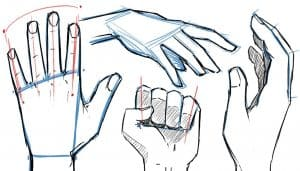# How to draw hands Step by Step

How to draw hands easy with this how-to video and step-by-step drawing instructions. Easy drawing tutorial for beginners and everyone.## You can refer to the simple step-by-step drawing guide below

### Step 1

First we will draw a rectangle to create the main part of the hand. Then divide the upper line of the trapezoid into four parts, to indicate the base of the fingers.

### Step 2

Next, we need to draw the fingers. To draw the fingers correctly, you should know that the length of the middle finger is equal to the length of the main part of the palm, which we drew in the previous step.

### Step 3

So let’s draw the fingers. And now look at the proportions of the other fingers, the index and ring fingers are slightly shorter than the middle finger. Also pay attention to the knuckles and their position. We have drawn three curves to indicate their location.

### Step 4

Next, we will draw the thumb. Next, we will start drawing the thumb. Note that the thumb sticks out unlike the other fingers. So let’s draw a triangle to indicate the base of the thumb.

### Step 5

Note that the thumb is slightly shorter and thicker than the rest of the fingers.

### Step 6

At this step we will add some details. Sketch the knuckles and nails. Draw the outer line of the palm, going from the base of the little finger downwards.

### Step 7

Erase the guides and add wrinkles and lines on the fingers.

Now you know the fundamentals of hand drawing. So let’s draw the hand from the opposite point of view.

First we draw the trapezoid.

As you can see, the drawing principles are exactly the same. Let’s draw the hand from the side.

First draw a rectangle (trapezoid from the side). Then add the fingers. Remove rules and add details.

When you draw the hand from the side, you should pay attention to the fingers. Note that the tops of the fingers are flat and the bottoms of the fingers are convex.

Since you learned how to draw hands, let’s start learning how to draw fists.

The fist is drawn in the same way as the open hand. First we draw the trapezoid.

Then outline the fingers and knuckles.

how to draw a fist

Draw thumbs.

how to draw a fist step by step